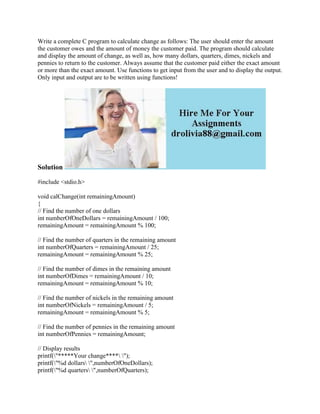Successfully reported this slideshow.

# Write a complete C program to calculate change as follows- The user s.docx×

# Write a complete C program to calculate change as follows- The user s.docx

Write a complete C program to calculate change as follows: The user should enter the amount the customer owes and the amount of money the customer paid. The program should calculate and display the amount of change, as well as, how many dollars, quarters, dimes, nickels and pennies to return to the customer. Always assume that the customer paid either the exact amount or more than the exact amount. Use functions to get input from the user and to display the output. Only input and output are to be written using functions!
Solution
#include <stdio.h>
void calChange(int remainingAmount)
{
// Find the number of one dollars
int numberOfOneDollars = remainingAmount / 100;
remainingAmount = remainingAmount % 100;
// Find the number of quarters in the remaining amount
int numberOfQuarters = remainingAmount / 25;
remainingAmount = remainingAmount % 25;
// Find the number of dimes in the remaining amount
int numberOfDimes = remainingAmount / 10;
remainingAmount = remainingAmount % 10;
// Find the number of nickels in the remaining amount
int numberOfNickels = remainingAmount / 5;
remainingAmount = remainingAmount % 5;
// Find the number of pennies in the remaining amount
int numberOfPennies = remainingAmount;
// Display results
printf(\"%d dollars\ \",numberOfOneDollars);
printf(\"%d quarters\ \",numberOfQuarters);
printf(\"%d dollars\ \",numberOfDimes);
printf(\"%d nickels\ \",numberOfNickels);
printf(\"%d pennies\ \",numberOfPennies);
}
int main()
{
float amt;
printf(\"Enter the amount in cents: \");
scanf(\"%f\",&amt);
int remainingAmount = (amt * 100);
calChange(remainingAmount);
return 0;
}
.

Write a complete C program to calculate change as follows: The user should enter the amount the customer owes and the amount of money the customer paid. The program should calculate and display the amount of change, as well as, how many dollars, quarters, dimes, nickels and pennies to return to the customer. Always assume that the customer paid either the exact amount or more than the exact amount. Use functions to get input from the user and to display the output. Only input and output are to be written using functions!
Solution
#include <stdio.h>
void calChange(int remainingAmount)
{
// Find the number of one dollars
int numberOfOneDollars = remainingAmount / 100;
remainingAmount = remainingAmount % 100;
// Find the number of quarters in the remaining amount
int numberOfQuarters = remainingAmount / 25;
remainingAmount = remainingAmount % 25;
// Find the number of dimes in the remaining amount
int numberOfDimes = remainingAmount / 10;
remainingAmount = remainingAmount % 10;
// Find the number of nickels in the remaining amount
int numberOfNickels = remainingAmount / 5;
remainingAmount = remainingAmount % 5;
// Find the number of pennies in the remaining amount
int numberOfPennies = remainingAmount;
// Display results
printf(\"%d dollars\ \",numberOfOneDollars);
printf(\"%d quarters\ \",numberOfQuarters);
printf(\"%d dollars\ \",numberOfDimes);
printf(\"%d nickels\ \",numberOfNickels);
printf(\"%d pennies\ \",numberOfPennies);
}
int main()
{
float amt;
printf(\"Enter the amount in cents: \");
scanf(\"%f\",&amt);
int remainingAmount = (amt * 100);
calChange(remainingAmount);
return 0;
}
.